# Types of triangles

A triangle is a three-sided polygon that has an interior angle at each of its vertices. Triangles are often classified by their angles or sides.

Triangle △ABC, shown above, has sides AB, BC, and AC, and angles A,B,and C, formed at vertices A, B, and C.

## Triangles classified by their angles

The following table shows the types of triangles classified by their angles.

TypeAnglesFigure
Acute all interior angles < 90°Obtuse 1 interior angle > 90°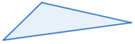Right 1 angle = 90°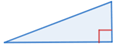Equiangular each interior angle = 60°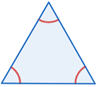## Triangles classified by their sides

The following table shows the types of triangles classified by their sides.

TypeSidesFigure
Scalene no 2 sides are congruentIsosceles 2 congruent sides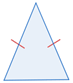Equilateral all sides are congruent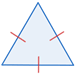## Using the Pythagorean Theorem to classify triangles by their angles

When one of the angles in a triangle is a right angle, the triangle is a right triangle. The lengths of the three sides of a right triangle follow Pythagorean Theorem. The Pythagorean Theorem can also be used to classifies triangles by angles as follows:

For △ABC, given that side c is the longest side:

• If c2 = a2 + b2, then △ABC is a right triangle with right angle C.
• If c2 > a2 + b2, then △ABC is an obtuse triangle with obtuse angle C.
• If c2 < a2 + b2, then △ABC is an acute triangle with all angles acute.

Example:

Classify a triangle that has side lengths 3, 6, and 8.

Since 8 is the largest side length, let c = 8, a = 3, and b = 6:

 c2 = 82 = 64 a2 + b2 = 32 +62 = 9 + 36 = 45

Since 64 > 45, c2 > a2 + b2, so the triangle is an obtuse triangle.